## Flip Flops-

Before you go through this article, make sure that you have gone through the previous article on Flip Flops.

We have discussed-

• A Flip Flop is a memory element that is capable of storing one bit of information.
• It is also called as Bistable Multivibrator since it has two stable states either 0 or 1.

There are following 4 basic types of flip flops-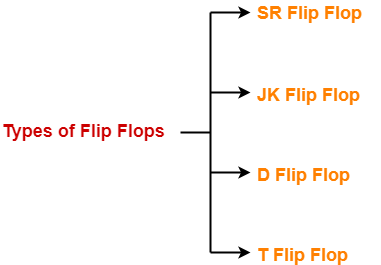1. SR Flip Flop
2. JK Flip Flop
3. D Flip Flop
4. T Flip Flop

## JK Flip Flop-

 JK flip flop is a refined & improved version of SR Flip Flopthat has been introduced to solve the problem of indeterminate statethat occurs in SR flip flop when both the inputs are 1.

In JK flip flop,

• Input J behaves like input S of SR flip flop which was meant to set the flip flop.
• Input K behaves like input R of SR flip flop which was meant to reset the flip flop.

## Construction of JK Flip Flop-

There are following two methods for constructing a JK flip flop-1. By using SR flip flop constructed from NOR latch
2. By using SR flip flop constructed from NAND latch

### 1. Construction of JK Flip Flop By Using SR Flip Flop Constructed From NOR Latch-

This method of constructing JK Flip Flop uses-

• SR Flip Flop constructed from NOR latch
• Two other connections

### Logic Circuit-

The logic circuit for JK Flip Flop constructed using SR Flip Flop constructed from NOR latch is as shown below-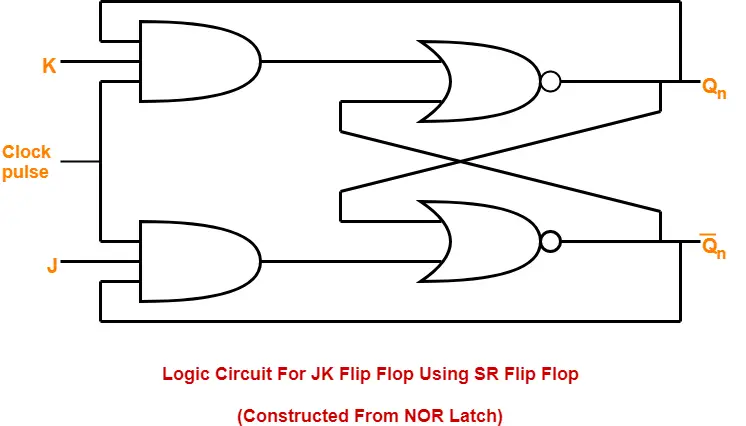### 2. Construction of JK Flip Flop By Using SR Flip Flop Constructed From NAND Latch-

This method of constructing JK Flip Flop uses-

• SR Flip Flop constructed from NAND latch
• Two other connections

### Logic Circuit-

The logic circuit for JK Flip Flop constructed using SR Flip Flop constructed from NAND latch is as shown below-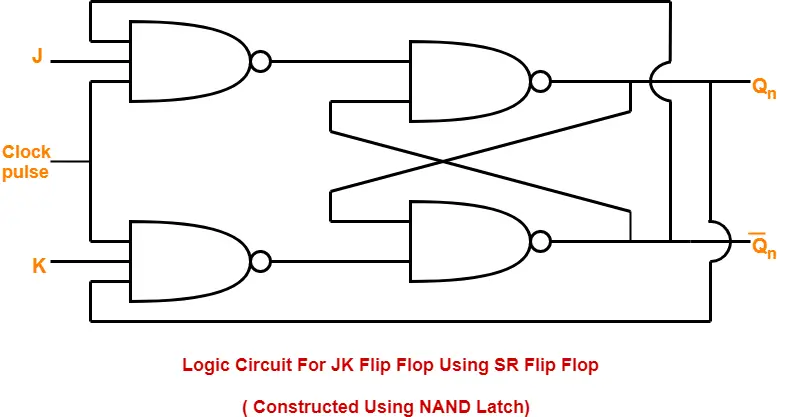### Logic Symbol-

The logic symbol for JK Flip Flop is as shown below-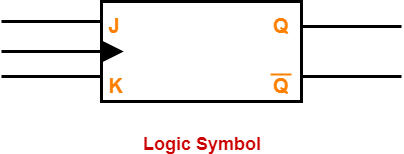### Truth Table-

The truth table for JK Flip Flop is as shown below-

 INPUTS OUTPUTS J K Qn (Present State) Qn+1 (Next State) 0 0 0 0 0 0 1 1 0 1 0 0 0 1 1 0 1 0 0 1 1 0 1 1 1 1 0 1 1 1 1 0

### Truth Table

The above truth table may be reduced as-

 INPUTS OUTPUTS REMARKS J K Qn (Present State) Qn+1 (Next State) States and Conditions 0 0 X Qn Hold State condition J = K = 0 0 1 X 0 Reset state condition J = 0 , K = 1 1 0 X 1 Set state condition J = 1 , K = 0 1 1 X Q’n Toggle state condition J = K = 1

### Characteristic Equation-

Draw a k map using the above truth table-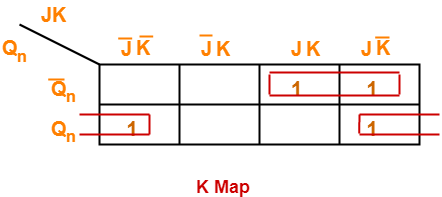From here-

Qn+1 = Q’n (JK + JK’) + Qn (J’K’ + JK’)

 Qn+1 = Q’nJ + QnK’

### Excitation Table-

The excitation table of any flip flop is drawn using its truth table.

 What is excitation table?For a given combination of present state Qn and next state Qn+1, excitation table tell the inputs required.

 Qn Qn+1 S R 0 0 0 X 0 1 1 X 1 0 X 1 1 1 X 0

## SR Flip Flop Vs JK Flip Flop-

Both JK flip flop and SR flip flop are functionally same.

The only difference between them is-

• In JK flip flop, indeterminate state does not occur.
• In JK flip flop, instead of indeterminate state, the present state toggles.
• In other words, the present state gets inverted when both the inputs are 1.

To gain better understanding about JK Flip Flop,

Watch this Video Lecture

Get more notes and other study material of Digital Design.

Watch video lectures by visiting our YouTube channel LearnVidFun.

## Flip Flops-

Before you go through this article, make sure that you have gone through the previous article on Flip Flops.

We have discussed-

• A Flip Flop is a memory element that is capable of storing one bit of information.
• It is also called as Bistable Multivibrator since it has two stable states either 0 or 1.

There are following 4 basic types of flip flops-1. SR Flip Flop
2. JK Flip Flop
3. D Flip Flop
4. T Flip Flop

## SR Flip Flop-

• SR flip flop is the simplest type of flip flops.
• It stands for Set Reset flip flop.
• It is a clocked flip flop.

## Construction of SR Flip Flop-

There are following two methods for constructing a SR flip flop-1. By using NOR latch
2. By using NAND latch

### 1. Construction of SR Flip Flop By Using NOR Latch-

This method of constructing SR Flip Flop uses-

• NOR latch
• Two AND gates

### Logic Circuit-

The logic circuit for SR Flip Flop constructed using NOR latch is as shown below-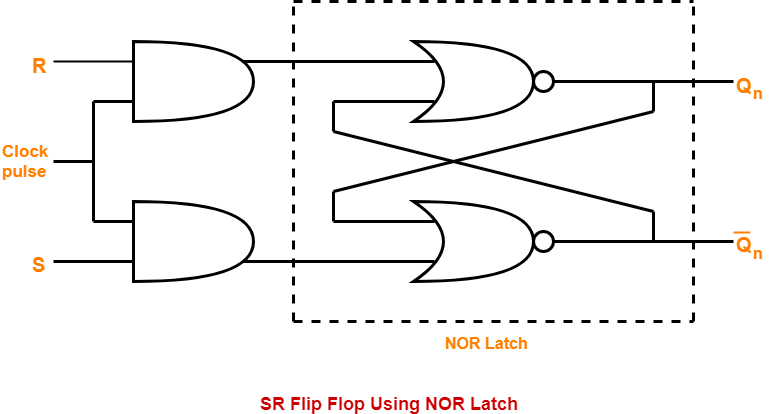### 2. Construction of SR Flip Flop By Using NAND Latch-

This method of constructing SR Flip Flop uses-

• NAND latch
• Two NAND gates

### Logic Circuit-

The logic circuit for SR Flip Flop constructed using NAND latch is as shown below-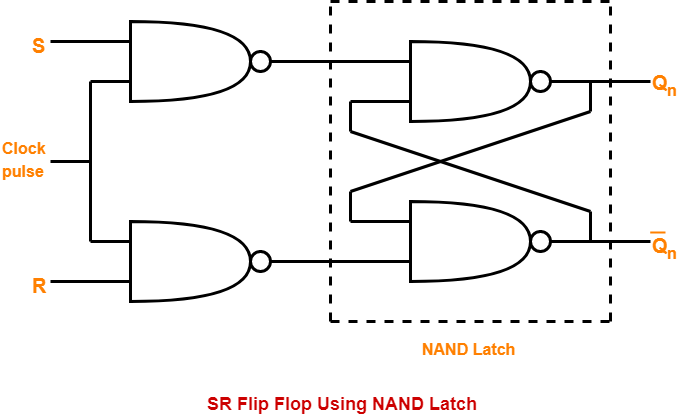### Logic Symbol-

The logic symbol for SR Flip Flop is as shown below-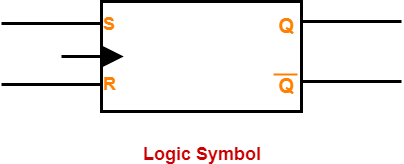### Truth Table-

The truth table for SR Flip Flop is as shown below-

 INPUTS OUTPUTS S R Qn (Present State) Qn+1 (Next State) 0 0 0 0 0 0 1 1 0 1 0 0 0 1 1 0 1 0 0 1 1 0 1 1 1 1 0 Indeterminate 1 1 1 Indeterminate

### Truth Table

The above truth table may be reduced as-

 INPUTS OUTPUTS REMARKS S R Qn (Present State) Qn+1 (Next State) States and Conditions 0 0 X Qn Hold State condition S = R = 0 0 1 X 0 Reset state condition S = 0 , R = 1 1 0 X 1 Set state condition S = 1 , R = 0 1 1 X Indeterminate Indeterminate state condition S = R = 1

### Characteristic Equation-

Draw a k map using the above truth table-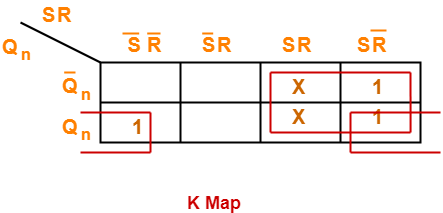From here-

Qn+1 = ( SR + SR’ ) ( Qn +  Q’n ) + Qn ( S’R’ + SR’ )

 Qn+1 = S + QnR’

### Excitation Table-

The excitation table of any flip flop is drawn using its truth table.

 What is excitation table?For a given combination of present state Qn and next state Qn+1, excitation table tell the inputs required.

 Qn Qn+1 S R 0 0 0 X 0 1 1 0 1 0 0 1 1 1 X 0

### Excitation Table

To gain better understanding about SR Flip Flop,

Watch this Video Lecture

Next Article- JK Flip Flop

Get more notes and other study material of Digital Design.

Watch video lectures by visiting our YouTube channel LearnVidFun.

## Flip Flop-

 A Flip Flop is a memory element that is capable of storing one bit of information.

A flip flop has two outputs as shown-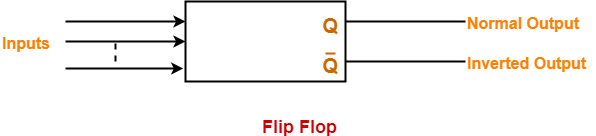A flip flop can maintain a binary state for an unlimited period of time as long as-

• Power is supplied to the circuit.
• Or until it is directed by an input signal to switch states.

A flip flop is also called as Bistable Multivibrator because it has two stable states either 0 or 1.

## Flip Flops Types-

Flip flops are of different types depending on how their inputs and clock pulses cause transition between two states.

There are 4 basic types of flip flops-1. SR Flip Flop
2. JK Flip Flop
3. D Flip Flop
4. T Flip Flop

We will discuss about these flip flops one by one.

To gain better understanding about Flip Flops in Digital Logic,

Watch this Video Lecture

Next Article- SR Flip Flop

Get more notes and other study material of Digital Design.

Watch video lectures by visiting our YouTube channel LearnVidFun.